# GCSE Maths Paper One (Foundation) Practice Paper in the Style of AQA - Non Calculator

In this assessment, students will be able to complete a timed GCSE Mathematics Paper 1 (Non-Calculator) in the style of AQA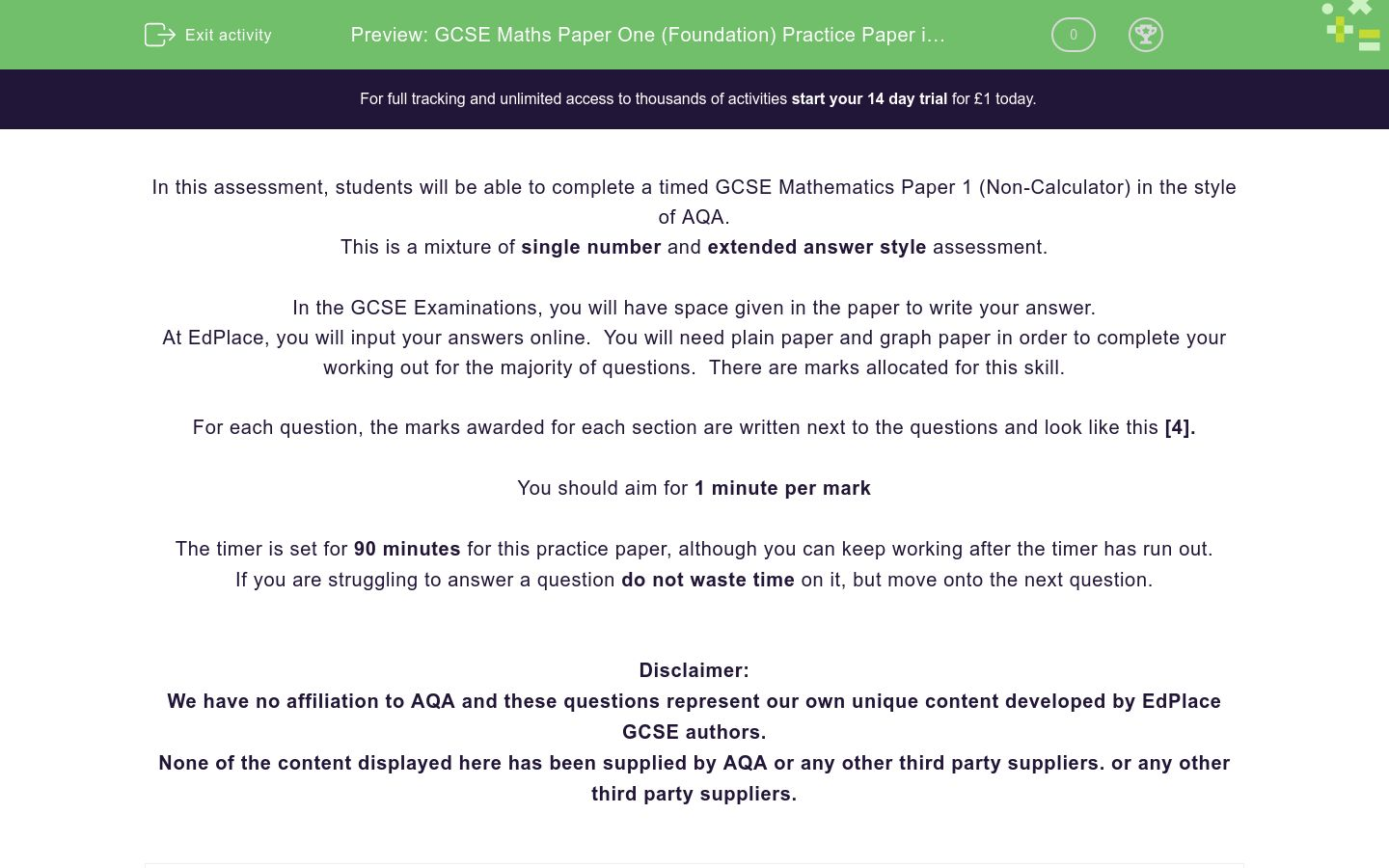Key stage:  KS 4

GCSE Subjects:   Maths

GCSE Boards:   AQA

Curriculum topic:   Foundation Practice Papers

Curriculum subtopic:   Non-Calculator Practice Papers

Difficulty level:### QUESTION 1 of 10

In this assessment, students will be able to complete a timed GCSE Mathematics Paper 1 (Non-Calculator) in the style of AQA.

This is a mixture of single number and extended answer style assessment.

In the GCSE Examinations, you will have space given in the paper to write your answer.

At EdPlace, you will input your answers online.  You will need plain paper and graph paper in order to complete your working out for the majority of questions.  There are marks allocated for this skill.

For each question, the marks awarded for each section are written next to the questions and look like this .

You should aim for 1 minute per mark

The timer is set for 90 minutes for this practice paper, although you can keep working after the timer has run out.

If you are struggling to answer a question do not waste time on it, but move onto the next question.

Disclaimer:

We have no affiliation to AQA and these questions represent our own unique content developed by EdPlace GCSE authors.

None of the content displayed here has been supplied by AQA or any other third party suppliers. or any other third party suppliers.

Write 0.62 as a percentage.



62%

6.2%

0.62%

31%

Which of these is a prime number between 10 and 20?



11

12

13

15

Work out 7 x (2+1)



15

21

16

14

What number is exactly half-way between 21 and 33?



25

26

27

28

Write 150 seconds in minutes(a) Write down the coordinates of point A.       

(b) (i) Plot the point with the coordinates (4,1). Label this point B     

(ii) Does point B lie on the line y = 3x – 2? Give a reason for your answer.      

(c) On the grid, draw the line y = 5      

Amrita has 3 dogs, each one eats ¾ of a tin of dog food each day.

She buys 10 tins of dog food.

Does she have enough to feed her dogs for 4 days?



(a) Work out(b) Work outStephen leaves home at 6 AM and cycles to work in half an hour at an average speed of 12 mph.

(a) Work out the distance Stephen cycled.       

Stephen worked for 9 hours and then cycled home. He took 50 minutes to cycle home.

(b) What time did Stephen get home?       

Kayliegh drives to a friend’s wedding in France with 3 friends.

The costs are…

£600 for 4 return ferry tickets

£650 to hire a villa to stay in

£200 for petrol for her car

The four friends split the cost equally. What is Kayliegh's share?



Here are some fractions.One of these fractions is not equal to 2/3.

Which fraction?



10/15

4/6

20/30

22/30

16/24

There are 30 pupils in a school class.

18 of the pupils are male

8 of the female students are right-handed

12 of the male students are left-handed.

(a) Use this information to complete the two-way table.      

 Male Female Total Left Handed Right Handed Total

One of the students is chosen at random.

(b) What is the probability that this is a left-handed girl?      

The total surface area of a cube is 150 cm2.

Find the volume.



James, John and Jim all earn the same each month.

James spends 65% of his salary and saves the rest.

John spends 34/50 of his salary and saves the rest.

Jim spends and saves his salary in the ratio 3:2

Work out who saves the least of their salary each month.



Here are a triangle and a rectangle.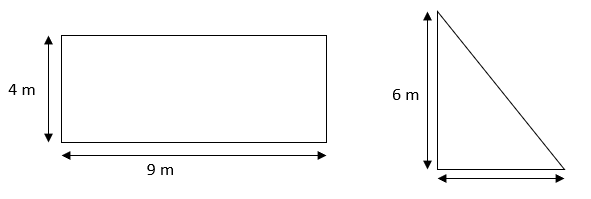The rectangle has an area three times bigger than the area of the triangle.

Work out the length of the base of the triangle.



s = ut + ½ at2

u = 3, t = 2 and a = 3

Find the value of s.



5 apples cost £1.55

3 apples and 4 oranges cost £2.41

Work out the cost of 2 apples and 3 oranges.



Eric wants to buy some Coffee.

The Food store and Caffeine Central both sell the same type of coffee.

He sees that each shop has the following special offers.

The Food Store.

"500g costs £ 3, 25% off the price"

Caffeine Central

"500g cost £3, Extra 25% for the same price"

Which jar of coffee is the best value?



Work outIn a bag, there are blue discs, red discs and black discs

There are three times as many blue discs as red discs

the number of blue discs: the number of black discs is 2:3

One disc is selected at random.

Work out the probability the disc is either blue or black



Work out 36.2 x 4.9



A Formula One car travels at 182.9 miles per hour.

Use approximations to estimate the of the number of miles travelled in 1 minute.



Solve the simultaneous equations

6x + 5y = 38

4x + 6y = 36



Here are two shapes, a rectangle and a triangle that have the same perimeter.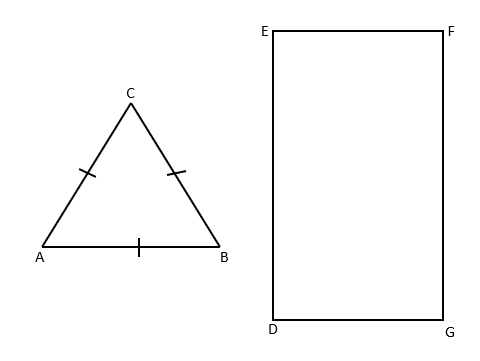FG = 10 cm

Area of DEFG = 50 cm2

Find the value of AB



a) Write down the exact value of sin 60.     

b) Here is a right-angled triangle.Cos 30 = 0.5

Find the value of x.         

The size of each interior angle of a regular polygon is 3 times the size of each exterior angle.

What is the name of this regular polygon?



• Question 1

Write 0.62 as a percentage.



62%
EDDIE SAYS
Nice and easy one to start with. Remember the rule that to change a decimal into a percentage, you just multiply it by 100.
• Question 2

Which of these is a prime number between 10 and 20?



11
13
EDDIE SAYS
A prime number is just a number that can only be divided by itself and 1. The only numbers that fit this description between 10 and 20 are 11,13,17 and 19
• Question 3

Work out 7 x (2+1)



21
EDDIE SAYS
A nice little BODMAS question. Did you spot the bracket? Remember this means you have to do this first.
• Question 4

What number is exactly half-way between 21 and 33?



27
EDDIE SAYS
There's a really easy way to do this. Add them together and then half it. Give it a try.
• Question 5

Write 150 seconds in minutes



2.5
EDDIE SAYS
The biggest mistake people make here is to put 2.30 Remember that 30 seconds is 0.5 minutes
• Question 6(a) Write down the coordinates of point A.       

(b) (i) Plot the point with the coordinates (4,1). Label this point B     

(ii) Does point B lie on the line y = 3x – 2? Give a reason for your answer.      

(c) On the grid, draw the line y = 5      

EDDIE SAYS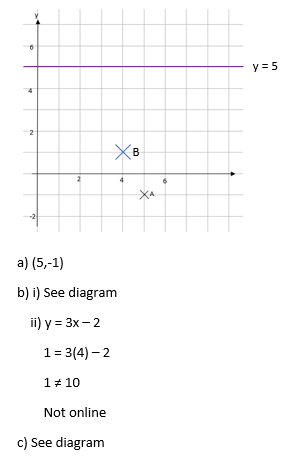• Question 7

Amrita has 3 dogs, each one eats ¾ of a tin of dog food each day.

She buys 10 tins of dog food.

Does she have enough to feed her dogs for 4 days?



EDDIE SAYS• Question 8

(a) Work out(b) Work outEDDIE SAYS• Question 9

Stephen leaves home at 6 AM and cycles to work in half an hour at an average speed of 12 mph.

(a) Work out the distance Stephen cycled.       

Stephen worked for 9 hours and then cycled home. He took 50 minutes to cycle home.

(b) What time did Stephen get home?       

EDDIE SAYS• Question 10

Kayliegh drives to a friend’s wedding in France with 3 friends.

The costs are…

£600 for 4 return ferry tickets

£650 to hire a villa to stay in

£200 for petrol for her car

The four friends split the cost equally. What is Kayliegh's share?



EDDIE SAYS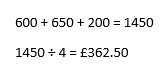• Question 11

Here are some fractions.One of these fractions is not equal to 2/3.

Which fraction?



22/30
EDDIE SAYS• Question 12

There are 30 pupils in a school class.

18 of the pupils are male

8 of the female students are right-handed

12 of the male students are left-handed.

(a) Use this information to complete the two-way table.      

 Male Female Total Left Handed Right Handed Total

One of the students is chosen at random.

(b) What is the probability that this is a left-handed girl?      

EDDIE SAYS• Question 13

The total surface area of a cube is 150 cm2.

Find the volume.



EDDIE SAYS• Question 14

James, John and Jim all earn the same each month.

James spends 65% of his salary and saves the rest.

John spends 34/50 of his salary and saves the rest.

Jim spends and saves his salary in the ratio 3:2

Work out who saves the least of their salary each month.



EDDIE SAYS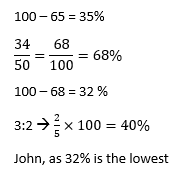• Question 15

Here are a triangle and a rectangle.The rectangle has an area three times bigger than the area of the triangle.

Work out the length of the base of the triangle.



EDDIE SAYS• Question 16

s = ut + ½ at2

u = 3, t = 2 and a = 3

Find the value of s.



EDDIE SAYS• Question 17

5 apples cost £1.55

3 apples and 4 oranges cost £2.41

Work out the cost of 2 apples and 3 oranges.



EDDIE SAYS• Question 18

Eric wants to buy some Coffee.

The Food store and Caffeine Central both sell the same type of coffee.

He sees that each shop has the following special offers.

The Food Store.

"500g costs £ 3, 25% off the price"

Caffeine Central

"500g cost £3, Extra 25% for the same price"

Which jar of coffee is the best value?



EDDIE SAYS• Question 19

Work outEDDIE SAYS• Question 20

In a bag, there are blue discs, red discs and black discs

There are three times as many blue discs as red discs

the number of blue discs: the number of black discs is 2:3

One disc is selected at random.

Work out the probability the disc is either blue or black



EDDIE SAYS• Question 21

Work out 36.2 x 4.9



EDDIE SAYS• Question 22

A Formula One car travels at 182.9 miles per hour.

Use approximations to estimate the of the number of miles travelled in 1 minute.



EDDIE SAYS• Question 23

Solve the simultaneous equations

6x + 5y = 38

4x + 6y = 36



EDDIE SAYS• Question 24

Here are two shapes, a rectangle and a triangle that have the same perimeter.FG = 10 cm

Area of DEFG = 50 cm2

Find the value of AB



EDDIE SAYS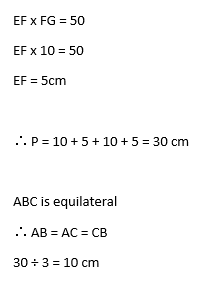• Question 25

a) Write down the exact value of sin 60.     

b) Here is a right-angled triangle.Cos 30 = 0.5

Find the value of x.         

EDDIE SAYS• Question 26

The size of each interior angle of a regular polygon is 3 times the size of each exterior angle.

What is the name of this regular polygon?



EDDIE SAYS